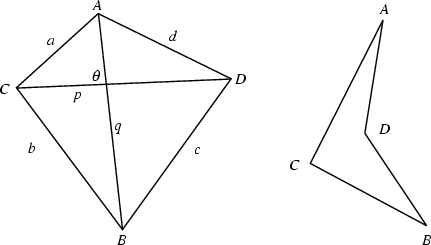A four-sided Polygon sometimes (but not very often) also known as a Tetragon. If not explicitly stated, all four Vertices are generally taken to lie in a Plane. If the points do not lie in a Plane, the quadrilateral is called a Skew Quadrilateral.

For a planar convex quadrilateral (left figure above), let the lengths of the sides be,,, and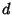, the Semiperimeter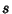, and the Diagonals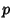and. The Diagonals are Perpendicular Iff. An equation for the sum of the squares of side lengths is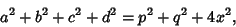(1)

whereis the length of the line joining the Midpoints of the Diagonals. The Area of a quadrilateral is given by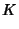(2)(3)(4)(5)

where (4) is known as Bretschneider's Formula (Beyer 1987).

A special type of quadrilateral is the Cyclic Quadrilateral, for which a Circle can be circumscribed so that it touches each Vertex. For Bicentric quadrilaterals, the Circumcircle and Incircle satisfy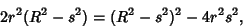(6)

whereis the Circumradius,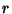in the Inradius, andis the separation of centers. A quadrilateral with two sides Parallel is called a Trapezoid.

There is a relationship between the six distances,,,,, and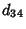between the four points of a quadrilateral (Weinberg 1972):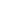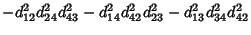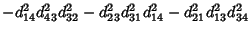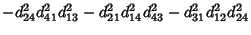(7)

References

Beyer, W. H. (Ed.) CRC Standard Mathematical Tables, 28th ed. Boca Raton, FL: CRC Press, p. 123, 1987.

Routh, E. J. Moment of Inertia of a Quadrilateral.'' Quart. J. Pure Appl. Math. 11, 109-110, 1871.

Weinberg, S. Gravitation and Cosmology: Principles and Applications of the General Theory of Relativity. New York: Wiley, p. 7, 1972.